Courses

# Test: Limit Of A Sum

## 10 Questions MCQ Test Mathematics For JEE | Test: Limit Of A Sum

Description
This mock test of Test: Limit Of A Sum for JEE helps you for every JEE entrance exam. This contains 10 Multiple Choice Questions for JEE Test: Limit Of A Sum (mcq) to study with solutions a complete question bank. The solved questions answers in this Test: Limit Of A Sum quiz give you a good mix of easy questions and tough questions. JEE students definitely take this Test: Limit Of A Sum exercise for a better result in the exam. You can find other Test: Limit Of A Sum extra questions, long questions & short questions for JEE on EduRev as well by searching above.
QUESTION: 1

Solution:
QUESTION: 2

### Express the shaded area in the form of an integral.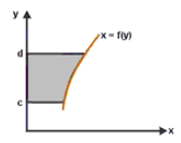Solution:

As the curve goes from c to d and the equation is x = f(y)
So the shaded area is ∫(c to d)f(y)dy

QUESTION: 3

### Evaluate as limit of  sum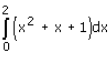Solution:

∫(0 to 2)(x2 + x + 1)dx
= (0 to 2) [x3/3 + x2/2 + x]½
= [8/3 + 4/2 + 2]
= 40/6
= 20/3

QUESTION: 4

Evaluate as limit of sum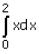Solution: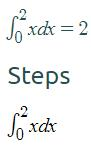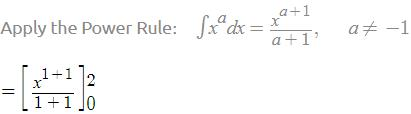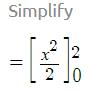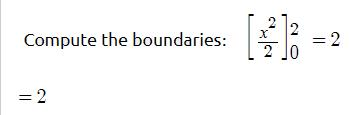QUESTION: 5

The value of definite integral depends on

Solution:
QUESTION: 6

Find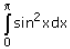Solution:

Using trigonometric identities, we have
cos2x=cos2x-sin2x  -(1) and cos2x+sin2x =1 -(2)
cos2x=1-sin2x , substituting this in equation (1) we get
cos2x=1-sin2x-sin2x=1-2sin2x
So,cos2x=1-2sin2x
2sin2x=1-cos2x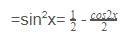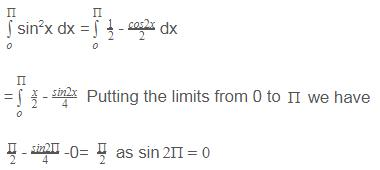QUESTION: 7

Evaluate as limit of  sum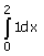Solution:
QUESTION: 8

The value of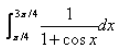is:​

Solution:
QUESTION: 9

Evaluate as limit of sum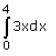Solution:

∫(0 to 4)3x dx
= [3x2/2] (0 to 4)
[3(4)2] / 2
= 24 sq unit

QUESTION: 10

The value of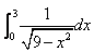is:

Solution:

∫(0 to 3)1/[(3)2 - (x)^2]½
∫1/[(a)2 - (x)2] = sin-1(x/a)
= [sin-1(x/3)](0 to 3)
= sin-1[3/3] - sin-1[0/3]
= sin-1
= π/2